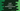# Swift 4 tutorial : Creating a multidimensional array### How to create multidimensional array in Swift 4 :

In this tutorial, we will learn how to create a multidimensional array in Swift 4. Multidimensional array means array of arrays. That is each element of an array is also an array. Let’s take a look how to create multidimensional array in swift :

### Creating an multidimensional array :

For one dimensional array, we use ’[]’ to create. For multidimensional array we can use ’[[]]‘. Following is a multidimensional array of integer arrays :

``[[1,2,3],[4,5,6],[7,8,9]]``

We can also assign it to a variable like :

``var myArray : [[Int]] = [[1,2,3],[4,5,6],[7,8,9]]``

### Iteration a swift multidimensional array :

Using two ‘for’ loops, we can iterate a multidimensional array. Example :

``````var myArray : [[Int]] = [[1,2,3],[4,5,6],[7,8,9]]

for first in myArray {
for second in first {
print("value \(second)")
}
}``````

Above example will print the following output :

``````value 1
value 2
value 3
value 4
value 5
value 6
value 7
value 8
value 9``````

### Adding new elements :

Since each element of a multidimensional array is also an array, we can access an element like ‘arrayName[index]’ and append values using ‘append()’ method. Not only ‘append’ , other array operations like ‘remove’,‘removeall’ etc. also applicable with multidimensional arrays.

``````var myArray : [[Int]] = [[1,2,3],[4,5,6],[7,8,9]]

myArray.append(10)

print (myArray)``````

This program will append ‘10 to the third element of ‘myArray’ i.e. ’[7,8,9]’. Output :

``[[1, 2, 3], [4, 5, 6], [7, 8, 9, 10]]``